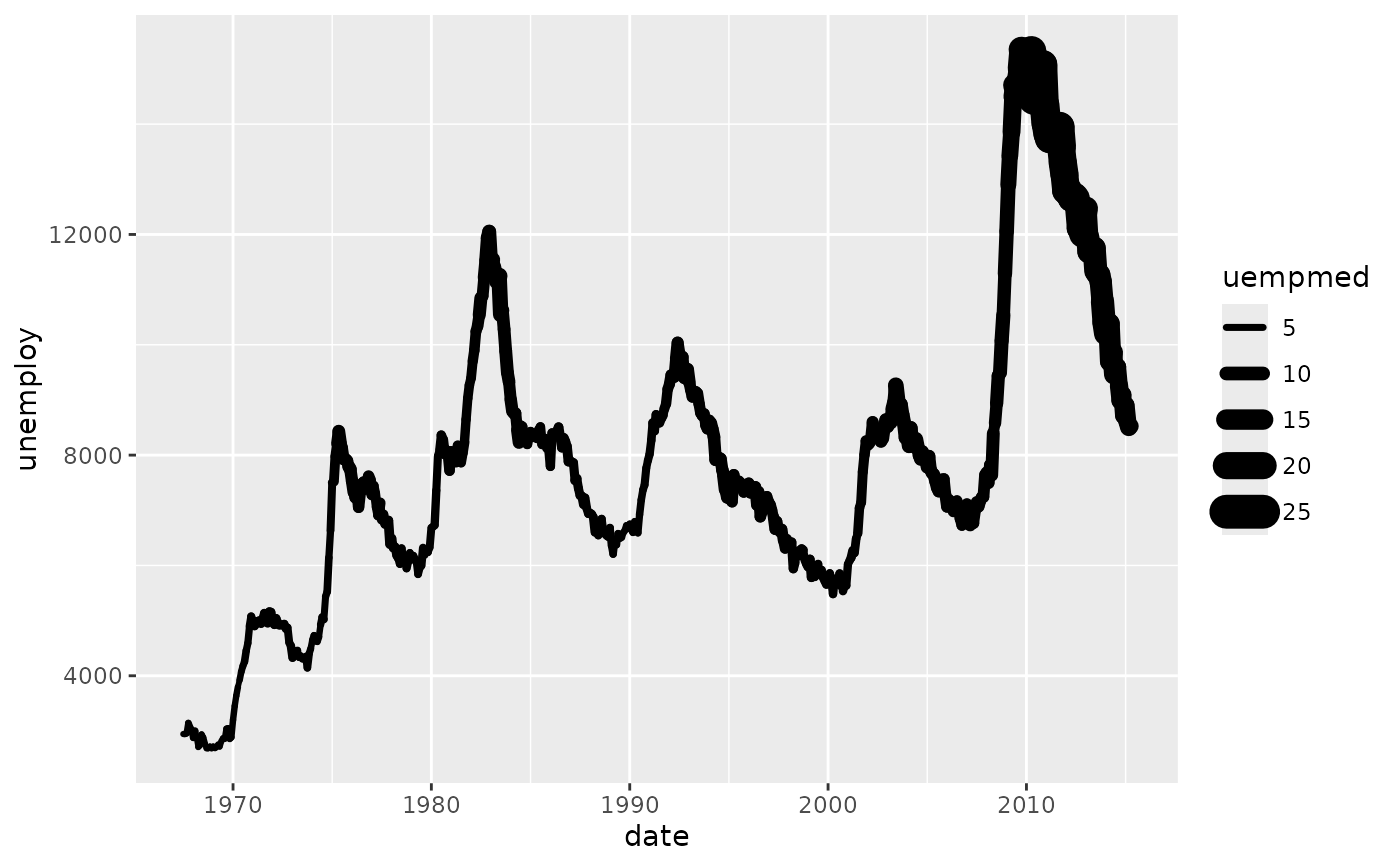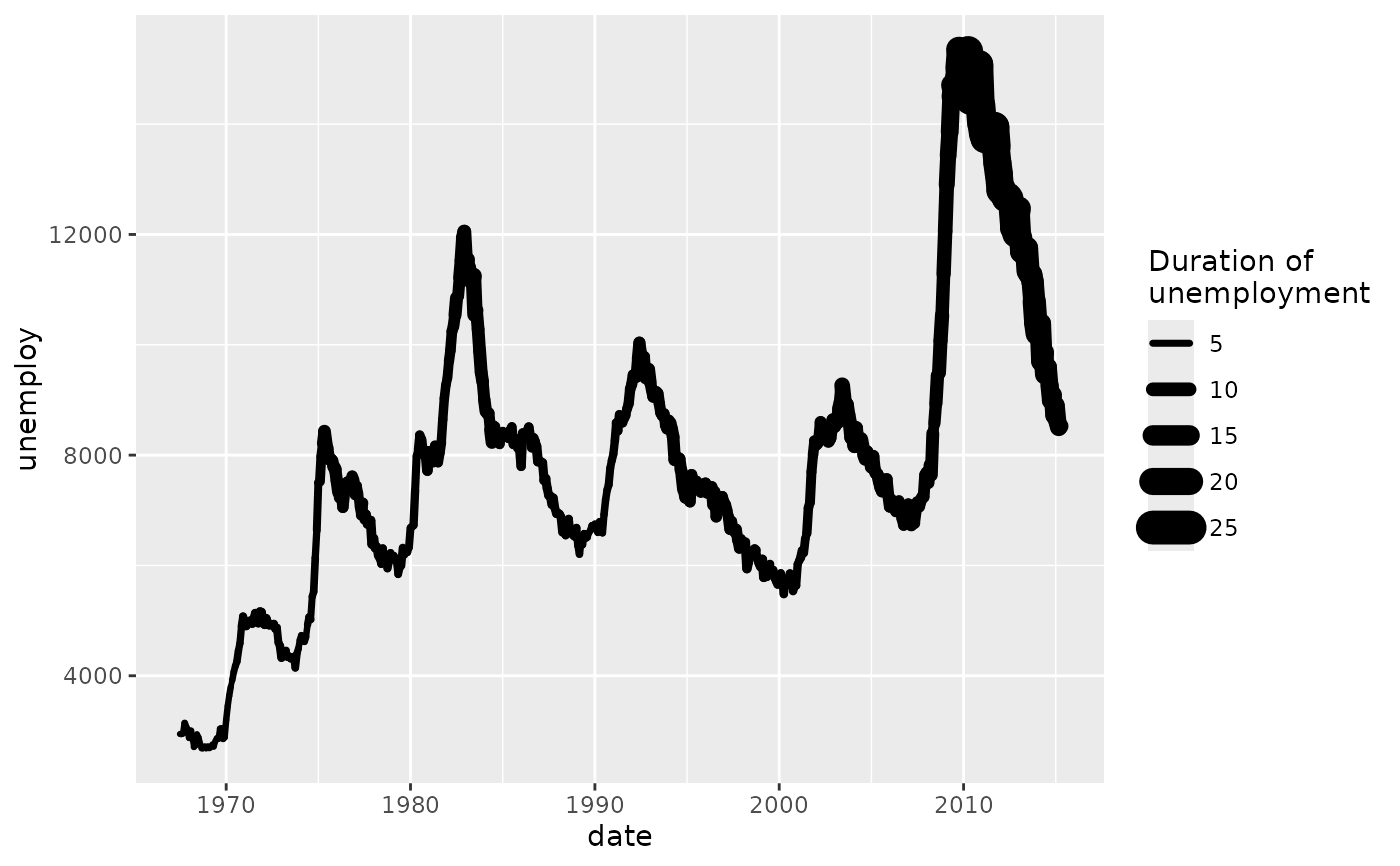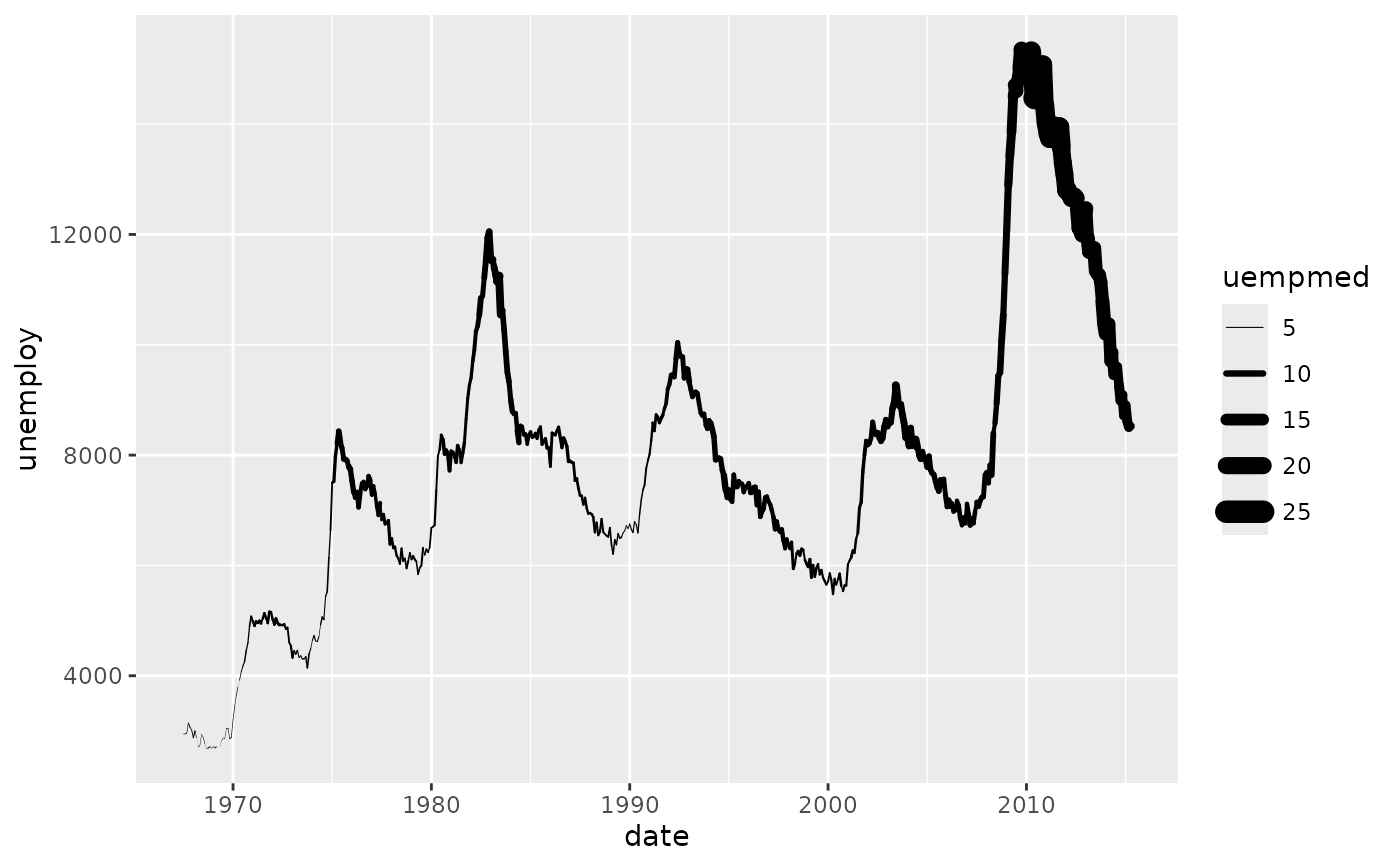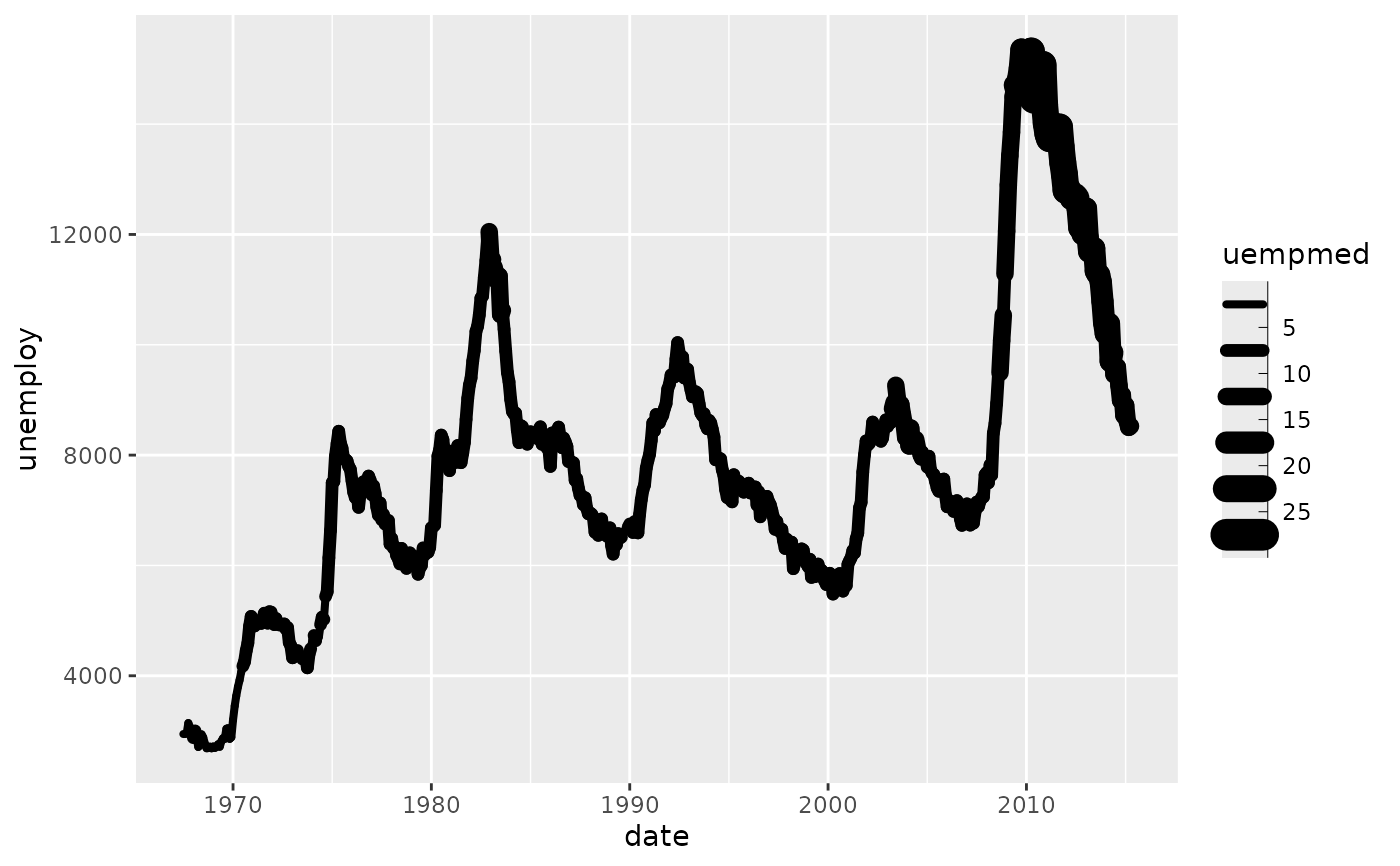scale_linewidth scales the width of lines and polygon strokes. Due to historical reasons, it is also possible to control this with the size aesthetic, but using linewidth is encourage to clearly differentiate area aesthetics from stroke width aesthetics.

## Usage

scale_linewidth(
name = waiver(),
breaks = waiver(),
labels = waiver(),
limits = NULL,
range = c(1, 6),
trans = "identity",
guide = "legend"
)

scale_linewidth_binned(
name = waiver(),
breaks = waiver(),
labels = waiver(),
limits = NULL,
range = c(1, 6),
n.breaks = NULL,
nice.breaks = TRUE,
trans = "identity",
guide = "bins"
)

## Arguments

name

The name of the scale. Used as the axis or legend title. If waiver(), the default, the name of the scale is taken from the first mapping used for that aesthetic. If NULL, the legend title will be omitted.

breaks

One of:

• NULL for no breaks

• waiver() for the default breaks computed by the transformation object

• A numeric vector of positions

• A function that takes the limits as input and returns breaks as output (e.g., a function returned by scales::extended_breaks()). Also accepts rlang lambda function notation.

labels

One of:

• NULL for no labels

• waiver() for the default labels computed by the transformation object

• A character vector giving labels (must be same length as breaks)

• An expression vector (must be the same length as breaks). See ?plotmath for details.

• A function that takes the breaks as input and returns labels as output. Also accepts rlang lambda function notation.

limits

One of:

• NULL to use the default scale range

• A numeric vector of length two providing limits of the scale. Use NA to refer to the existing minimum or maximum

• A function that accepts the existing (automatic) limits and returns new limits. Also accepts rlang lambda function notation. Note that setting limits on positional scales will remove data outside of the limits. If the purpose is to zoom, use the limit argument in the coordinate system (see coord_cartesian()).

range

a numeric vector of length 2 that specifies the minimum and maximum size of the plotting symbol after transformation.

trans

For continuous scales, the name of a transformation object or the object itself. Built-in transformations include "asn", "atanh", "boxcox", "date", "exp", "hms", "identity", "log", "log10", "log1p", "log2", "logit", "modulus", "probability", "probit", "pseudo_log", "reciprocal", "reverse", "sqrt" and "time".

A transformation object bundles together a transform, its inverse, and methods for generating breaks and labels. Transformation objects are defined in the scales package, and are called <name>_trans (e.g., scales::boxcox_trans()). You can create your own transformation with scales::trans_new().

guide

A function used to create a guide or its name. See guides() for more information.

n.breaks

An integer guiding the number of major breaks. The algorithm may choose a slightly different number to ensure nice break labels. Will only have an effect if breaks = waiver(). Use NULL to use the default number of breaks given by the transformation.

nice.breaks

Logical. Should breaks be attempted placed at nice values instead of exactly evenly spaced between the limits. If TRUE (default) the scale will ask the transformation object to create breaks, and this may result in a different number of breaks than requested. Ignored if breaks are given explicitly.

## Examples

p <- ggplot(economics, aes(date, unemploy, linewidth = uempmed)) +
geom_line(lineend = "round")
pp + scale_linewidth("Duration of\nunemployment")p + scale_linewidth(range = c(0, 4))# Binning can sometimes make it easier to match the scaled data to the legend
p + scale_linewidth_binned()